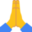# B4J Question[BANano] Any possibility of function overload like?

#### Mashiane

##### Expert
Longtime User
Hi there

In VB there was function overload and I believe java has something like that too, ie defining a function multiple times but with different initialization parameters. Well, this is necessary it, but something interesting, I have just came across.

B4X:
``````function sum() {
let result = 0;
for (const argument of arguments) {
result += argument;
}
return result;
}

sum(5, 8); // 13``````

Which is valid javacript. I'm curious...

Solution
Sorry, I should have used your example:

Variable Arguments, Take Two:
``````Sub sum(A() As Object) As Object

If A.Length = 0 Then
Return Null
Else If A(0) Is Int Then
Dim IntSum As Int = 0
For Each I As Int In A
IntSum = IntSum + I
Next
Return IntSum
else if A(0) Is Float Or A(0) Is Double Then
Dim DoubleSum As Double = 0
For Each D As Double In A
DoubleSum = DoubleSum + D
Next
Return DoubleSum
else if A(0) Is String Then
Dim StringSum As String = ""
For Each S As String In A
StringSum = StringSum & S
Next
Return StringSum
Else
Return Null
End If

End Sub

Log(sum(Array As Object(5, 8)))...``````

#### emexes

##### Expert
This might be enough to sate your curiousity:

Variable Arguments (good as):
``````Sub FunctionWithVariableArguments(A() As Object)
Log("There are " & A.Length & " arguments")

For I = 0 To A.Length - 1
Dim ShowAs As String = A(I)
If A(I) Is Int Then
ShowAs = "the integer " & ShowAs
else if A(I) Is String Then
ShowAs = "the string """ & ShowAs & """"
End If

Log(I & " = " & ShowAs)
Next
End Sub

FunctionWithVariableArguments(Array As Object(3, "point", 1, 4, 1, 5, 9, "etc", 2.718))``````

Log Output:
``````Waiting for debugger to connect...
Program started.
There are 9 arguments
0 = the integer 3
1 = the string "point"
2 = the integer 1
3 = the integer 4
4 = the integer 1
5 = the integer 5
6 = the integer 9
7 = the string "etc"
8 = 2.718``````

•Mashiane

#### alwaysbusy

##### Expert
Longtime User
Which is valid javacript
Correct, but it's not valid B4X syntax so you have indeed have to use a trick as described above. That is why many BANano methods also have an Array as parameter.

Alwaysbusy

#### emexes

##### Expert
Sorry, I should have used your example:

Variable Arguments, Take Two:
``````Sub sum(A() As Object) As Object

If A.Length = 0 Then
Return Null
Else If A(0) Is Int Then
Dim IntSum As Int = 0
For Each I As Int In A
IntSum = IntSum + I
Next
Return IntSum
else if A(0) Is Float Or A(0) Is Double Then
Dim DoubleSum As Double = 0
For Each D As Double In A
DoubleSum = DoubleSum + D
Next
Return DoubleSum
else if A(0) Is String Then
Dim StringSum As String = ""
For Each S As String In A
StringSum = StringSum & S
Next
Return StringSum
Else
Return Null
End If

End Sub

Log(sum(Array As Object(5, 8)))    '13

Log(sum(Array As Object("5", "8")))    '58``````

Log output:
``````Waiting for debugger to connect...
Program started.
13
58``````

•Mashiane and alwaysbusy

#### emexes

##### Expert
That is why many BANano methods also have an Array as parameter.

yes, it'd be nice if declared argument "..." would magically wrap the remaining arguments like "Array As Object( ... )"

on the other hand, that's just yet another special case that risks complicating B4X parsing down the track

vs after you've used "Array As Object" a few times, the verbiage blends into the background and looks "normal"

•alwaysbusy

#### Toky Olivier

##### Active Member
Longtime User
but it's not valid B4X syntax
Yes, like some OOP features we may need...
Anyway, thank you for your tips.